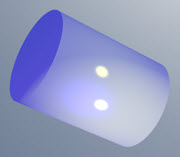# Cylinders - Overview

Author:
Christine
Topic:
Cylinder

## Cylinders - Overview

Cylinder Facts:Notice these interesting things:
• It has a flat base and a flat top
• The base is the same as the top
• From base to top the shape stays the same
• It has one curved side
• The cross-section of a cylinder is a circleAn object shaped like a cylinder is said to be cylindrical.Surface Area of a Cylinder: The Surface Area has these parts:
• Surface Area of Both Ends = 2 × π × r2
• Surface Area of Side = 2 × π × r × h
Which together make: Surface Area = 2 × π × r × (r+h) Example: h = 7 and r = 2 Surface Area= 2 × π × r × (r+h)   ﻿ ﻿ = 2 × π × 2 × (2+7) ﻿ ﻿  = 2 × π × 2 × 9   ﻿ ﻿ = 36 π   ﻿ ﻿ ≈ 113.097 Volume of a Cylinder: To calculate the volume we multiply the area of the base by the height of the cylinder:
• Area of the base: π × r2
• Height: h
And we get: Volume = π × r× h Example: h = 7 and r = 2 Volume= π × r2 × h   ﻿ = π × 22 × 7   ﻿ = 28 π   ﻿ ≈  87.96 Volume = pizza How to remember: Volume = pizzaImagine you just cooked a pizza. The radius is "z", and the thickness "a" is the same everywhere ... what is the volume? Volume =   pi × z × z × a (we would normally write "pi" as π, and z × z as z2, but you get the idea!)